# Is Methylamine (CH3NH2) an acid or base? Weak or Strong - Conjugate acid, base pairs

Home  > Chemistry Article > Is CH3NH2 an acid or base?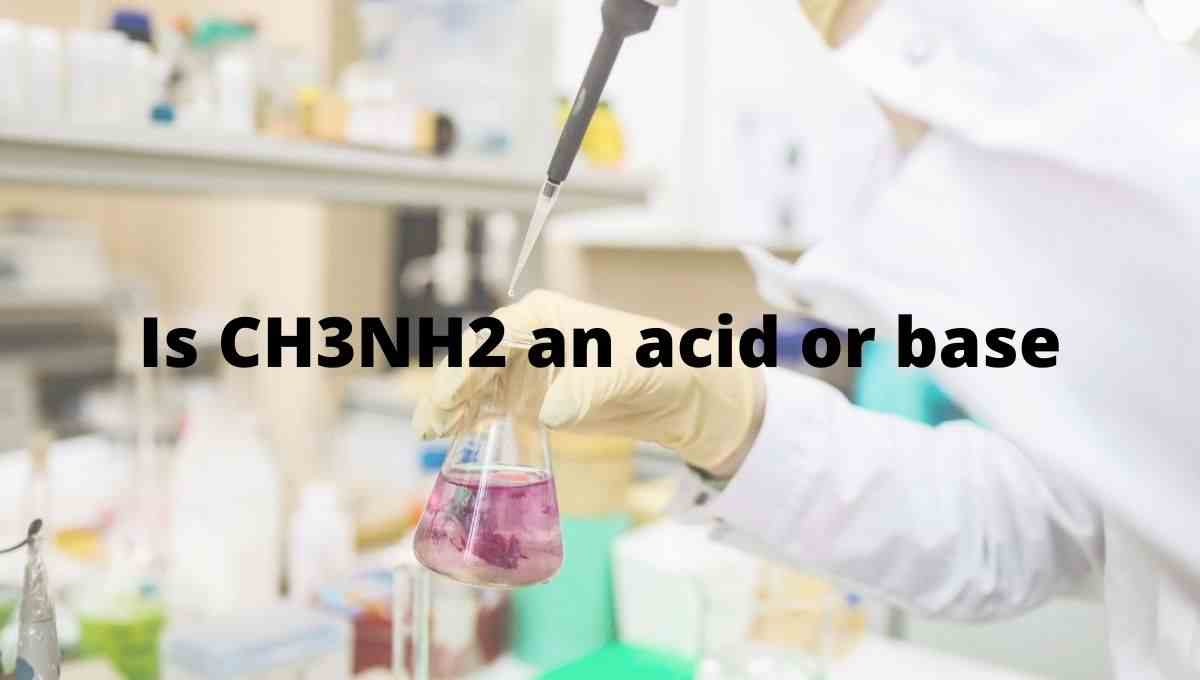Methylamine appears as colorless gas and has a fishy, ammoniacal odor having the chemical formula CH3NH2. It is a derivative of ammonia but one hydrogen atom of ammonia is being replaced by a methyl group(CH3).

In this article, we will discuss Is CH3NH2 acid or base? Is it strong or weak? Its conjugate acid-base pairs, etc.

So, Is CH3NH2 an acid or base? CH3NH2 is an base in nature as when dissolved in an aqueous solution, it accepts the H+ ion from a water molecule and produces hydroxide ions (OH) that correspondingly show its basic nature.

 Name of Molecule Methylamine Chemical formula CH3NH2 Conjugate acid CH3NH3+ Nature Weak base Base dissociation constant (Kb) 4.7 × 10-4
Page Contents

## Why CH3NH2 is a base?

A base is defined as a proton acceptor or lone pair donor. When CH3NH2 dissolves in water, it will accept the H+ ion from the water and gets converted into conjugate acid(CH3NH3+) and produces hydroxide ions(OH).

⇒ CH3NH2+ H2O  ⇌  CH3NH3++ OH

The production of hydroxide ions on dissolving in an aqueous solution shows the basic nature of CH3NH2.

Theoretically, we have two important acid-base theories to know whether CH3NH2 is acid or base.

(a). Arrhenius theory

(b). Bronsted-Lowry theory

(1). Arrhenius theory:

According to Arrhenius theory, the compound is said to be Arrhenius base when it produces OH ion through ionization or through dissociation in water and increases the concentration of OH ions in an aqueous solution. (first definition)

Or a compound is said to be Arrhenius base when substance contains at least one unit of OH in the chemical formula and produces OH ions in water. (second definition)

Clearly, when CH3NH2 is dissolved in an aqueous solution it accepts the proton and produces OH ion, and from the point of first Arrhenius definition, CH3NH2 will act as Arrhenius base as it is able to increase the concentration of OH- in the final solution.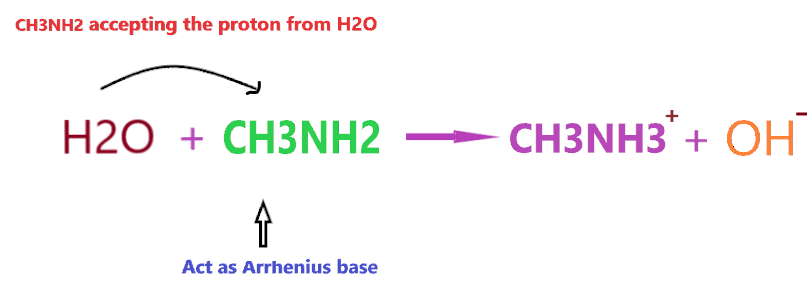However, if you consider, 2nd definition of Arrhenius base then CH3NH2 will not act as Arrhenius base because it doesn’t contain any OH in its chemical formula.

(2). Bronsted-Lowry concept:

According to the Bronsted-Lowry concept, a compound is said to be base when it accepts the proton from other compounds and forms the conjugate acid. Or you can say proton acceptor compounds are classified as Bronsted-Lowry base.

Take an example to understand whether CH3NH2 base or acid according to the Bronsted-lowry theory-

Consider the reaction of CH3NHwith HCl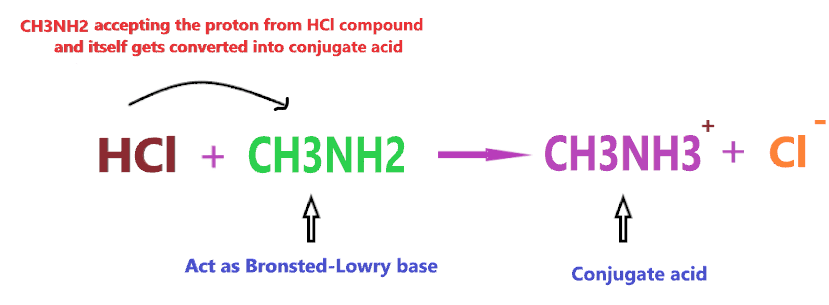According to the above reaction, when CH3NH2 reacts with a strong acid(HCl), then it will accept the one proton from HCl and itself gets converted into conjugate acid(CH3NH3+), hence, according to the above definition, CH3NH2 will act as Bronsted-Lowry base.

## Is CH3NH2 strong base or weak base?

To know whether CH3NH2 is a strong base or weak, you must know the basic difference between a strong base or a weak base.

Strong base: A compound is a strong base when it completely dissociates in an aqueous solution and liberates a large number of hydroxide ions. All moles of the strong base dissociate into hydroxide ion(OH) and no part remains undissociated into the solution.

Example: Sodium hydroxide(NaOH), Barium hydroxide (Ba(OH)2), Calcium hydroxide (Ca(OH)2), Lithium hydroxide (LiOH), Potassium hydroxide (KOH), etc.

Weak base: A compound is a weak base when it partially or not completely dissociate in an aqueous solution. It means only some parts of the weak base dissociates in the solution to produce OH ion but some parts remain undissociated inside the solution.

Example- Ammonia hydroxide (NH4OH), Ammonia (NH3), etc.

So, Is Methylamine (CH3NH2) a strong base or weak base? CH3NH2 is considered a weak base because when it is dissolved in an aqueous solution then not all the molecules of it react with water to yield OH ions, very few molecules of CH3NH2 react with water molecule ions and produce OH ion in the solution.

And the amount of OH produced in an aqueous solution is very low as compared to the number of CH3NH2 moles we dissolved in the solution.As shown in the figure, when CH3NH2 is dissolved in water, it accepts the hydrogen ion from the water molecule and produces two ions(CH3NH3+ and OH) but the ion(CH3NH3+) is not stable in an alkaline environment, it keeps breaking into CH3NH2 and H+.

⇒ CH3NH3⇔ CH3NH+ H+

Hence, not all the CH3NH2 molecules react with water ions and produce OH ions, most of them stay together, only, a few molecules do interact with water, therefore, CH3NH2 is considered a weak base in nature.

Let’s understand why CH3NH2 acts as the weak base with the help of the dissociation constant value concept.

⇒ If the value of the dissociation constant of acid is greater than 1 (Ka > 1), then the nature of the compound is a strong acid.

If Ka < 1, then the nature of the compound is a weak acid.

If Kb >1, then the nature of the compound is a strong base.

If Kb <1, then the nature of the compound is a weak base.

The base dissociation constant value(Kb) for CH3NH2 is 4.7 × 10-4 that’s way lower than recommended value for the Strong base, hence, CH3NH2 is a weak base in nature.

Here’s the list of some common acids and bases with their strength.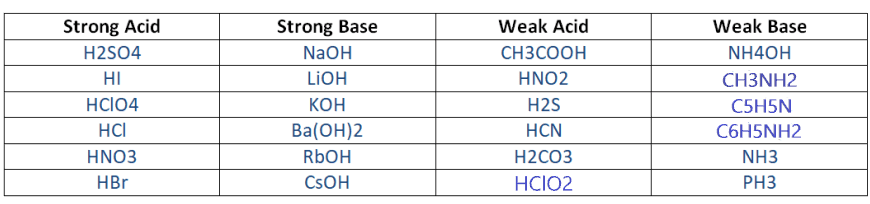## Why CH3NH2 act as a Lewis base?

Lewis’s theory is a very important acid-base theory to check whether a compound (CH3NH2) is acid or base?

According to the Lewis theory, a compound is said to be acid when it accepts the pair of electrons and a compound is said to be base when it donates the pair of electrons.

Lewis acid → lone pair acceptor

Lewis base → lone pair donator

Now CH3NH2 acts as lewis’s base because the central nitrogen atom has two lone pairs of electrons and it is always ready to give up the lone pair of electrons to another compound as it is very less electronegative in nature.

Let’s understand it with the help of an example-The electrons in the hydrogen-chlorine bonds attracted towards the chloride ion because of it high electronegativity leaving hydrogen slightly positive and chlorine negative.

The lone pair on the nitrogen of CH3NH2 molecule attracted towards hydrogen atom in HCl molecule.

As it approaches it, the electrons in the hydrogen-chlorine bond are repelled still further towards the chlorine.

Eventually, a co-ordinate bond is formed between the nitrogen and the hydrogen, and the chlorine breaks away as a chloride ion.

The whole HCl molecule acts as Lewis acid as it accept the lone pair from nitrogen atom, and in this process it breaks up. [Lewis acid & base guide here)

So, HCl accepts the lone pair of the electron, therefore, it is Lewis acid and CH3NH2 donates the lone pair of the electron, therefore, it is Lewis base.

## What is the conjugate acid of CH3NH2?

In technical terms, Compounds differentiated from each other by a single proton(H+) are said to be Conjugate acid-base pairs.

In simple terms, when the proton is removed from parent acid then the compound is formed which is called the conjugate base of that acid and when the proton is added to the parent base then the compound is formed which is called conjugate acid of that base.

Concept of Conjugate acid-base pair-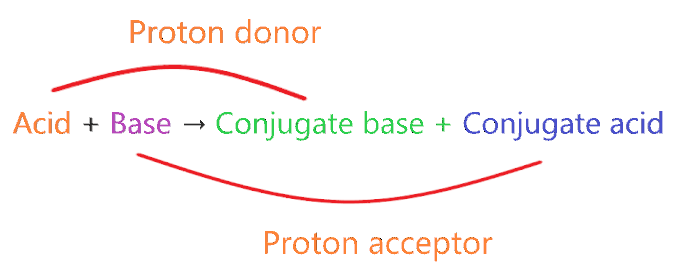• A very weak acid forms the strong conjugate base.
• A very weak base forms strong conjugate acid.
• A very strong acid forms the weak conjugate base.
• A very strong base forms weak conjugate acid.

⇒ Very weak means it doesn’t act as acid or base when dissolved in an aqueous solution.

⇒ Very strong means, acid or base ionizes 100% when dissolved in an aqueous solution.

So, what is the conjugate acid of CH3NH2As we discussed earlier, CH3NH2 is a weak base, hence, it will form a conjugate acid by adding one proton to itself.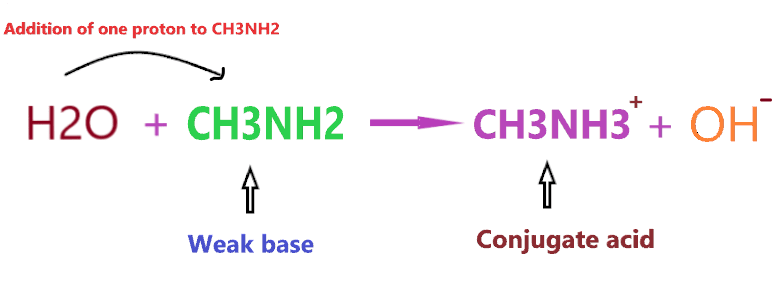As you see in the above reaction, CH3NH2 is a weak base and we know a weak base always forms a conjugate acid(not necessarily the strong one).

So, CH3NH3+ is the conjugate acid of CH3NH2.

## Summary

• Is CH3NH2 an acid or base? CH3NH2 is considered as a base in nature because the nitrogen atom consists of one lone pair which can be used to accept the proton, hence, this leads to the formation of CH3NH3+ and OH when it is dissolved in water. The presence of OH in the aqueous solution results in pH increasing and this makes the aqueous solution of CH3NH2 basic in nature.
• Methylamine (CH3NH2) is considered a weak base because not all the molecules of it react with water ions and produce OH ions, most of them stay together, only, a few molecules do interact with water, Therefore, the amount of OH ions produced in an aqueous solution is very low as compared to the number of CH3NH2 moles we dissolved in the solution.
• The base dissociation constant value for CH3NH2 is approximately 4.7 × 10-4 which is less than 1 and that shows its weak base nature.
• The conjugate acid of CH3NH2 is Methylammonium ion (CH3NH3+)
##### Subscribe to Blog via Email

Join 2 other subscribers

Share it...